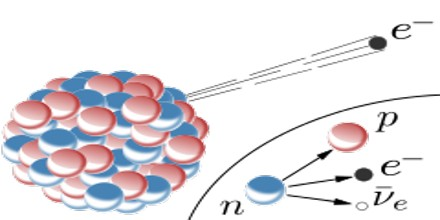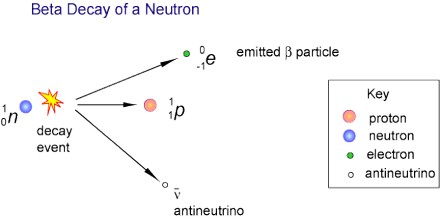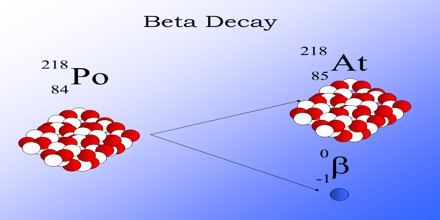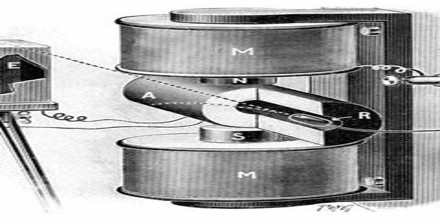About Beta Decay

Definition

Beta Decay is a radioactive process in which an electron is emitted from the nucleus of a radioactive atom, along with an unusual particle called an antineutrino. The neutrino is an almost massless particle that carries away some of the energy from the decay process. Because this electron is from the nucleus of the atom, it is called a beta particle to distinguish it from the electrons which orbit the atom.

Beta Decay occurs when an atom has either too many protons or too many neutrons in its nucleus. Two types of beta decay can occur. One type (positive beta decay) releases a positively charged beta particle called a positron, and a neutrino; the other type (negative beta decay) releases a negatively charged beta particle called an electron, and an antineutrino. The neutrino and the antineutrino are high energy elementary particles with little or no mass and are released in order to conserve energy during the decay process. Negative beta decay is far more common than positive beta decay.Beta Decay was named (1899) by Ernest Rutherford when he observed that radioactivity was not a simple phenomenon. He called the less penetrating rays alpha and the more penetrating rays beta. Most beta particles are ejected at speeds approaching that of light.

It is a consequence of the weak force, which is characterized by relatively lengthy decay times. Nucleons are composed of up or down quarks, and the weak force allows a quark to change type by the exchange of a W boson and the creation of an electron/antineutrino or positron/neutrino pair. For example: a neutron, composed of two down quarks and an up quark, decays to a proton composed of a down quark and two up quarks. Decay times for many nuclides that are subject to beta decay can be thousands of years.

Beta Decay conserves a quantum number known as the lepton number, or the number of electrons and their associated neutrinos, other leptons are the muon and tau particles. These particles have lepton number +1, while their antiparticles have lepton number −1. Since a proton or neutron has lepton number zero, β+ decay (a positron, or antielectron) must be accompanied with an electron neutrino, while β− decay (an electron) must be accompanied by an electron antineutrino.Neutrinos

The study of beta decay provided the first physical evidence for the existence of the neutrino. In both alpha and gamma decay, the resulting particle has a narrow energy distribution, since the particle carries the energy from the difference between the initial and final nuclear states. The distribution of beta particle energies was in apparent contradiction to the law of conservation of energy. If beta decay were simply electron emission as assumed at the time, then the energy of the emitted electron should have a particular, well-defined value. For beta decay, however, the observed broad distribution of energies suggested that energy is lost in the beta decay process. This spectrum was puzzling for many years.Nuclear Transmutation

If the proton and neutron are part of an atomic nucleus, the above described decay processes transmute one chemical element into another. Beta decay does not change the number (A) of nucleons in the nucleus, but changes only its charge Z. Thus the set of all nuclides with the same A can be introduced; these isobaric nuclides may turn into each other via beta decay. For a given A there is one that is most stable. It is said to be beta stable, because it presents a local minima of the mass excess: if such a nucleus has (A, Z) numbers, the neighbour nuclei (A, Z−1) and (A, Z+1) have higher mass excess and can beta decay into (A, Z), but not vice versa. For all odd mass numbers A, there is only one known beta-stable isobar. For even A, there are up to three different beta-stable isobars experimentally known.

Uses of Beta Rays

Beta rays, also known as beta particles, are used in many applications in a wide range of fields.

Uses in Medicine: Radioisotopes chemicals that emit radiation are widely used in medicine. In a process known as brachytherapy, beta radioisotopes can be used to irradiate areas inside a patient to prevent the growth of certain tissues. This approach has been used successfully to prevent the clogging of arterial inserts called stents. Beta particles are also used in some forms of therapy to kill cancer cells. In addition, the emission of beta particles is used indirectly in the medical scanning technique known as positron emission tomography (PET).Uses in Industry: Beta rays have a number of important uses in industrial processes. Since they can pass through some materials, they are used to gauge the thickness of films of material coming off production lines such as paper and plastic film. A similar process checks the integrity of sewn seams in textiles. In another application, the thickness of various coatings, such as paints, can be deduced from the amount of beta particles scattered back from that surface.

Tracers: Radioisotopes are commonly used as tracers in chemical and biological research. By synthesizing molecules containing a radioactive atom, the path and fate of that type of molecule in a particular reaction or metabolic process can be followed by tracking the radioactive signal of the isotope. One radioisotope used for this process is carbon-14 which can be inserted into organic or biological molecules and followed by its beta radiation signal.